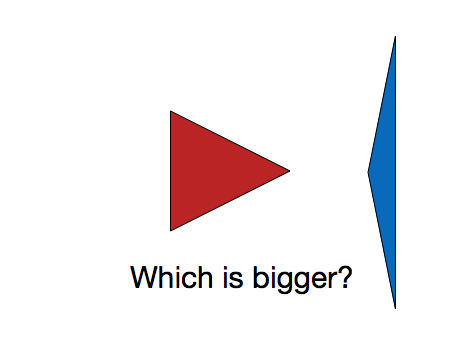# Triangle Ratios

Geometry Level 1Let $A$ be the area of a triangle of side lengths $5$, $5$ and $6$ and let $B$ be the area of a triangle of side lengths $5$, $5$ and $8$. What is $A/B$?

×